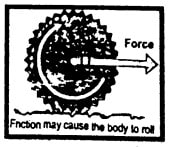Why rolling friction is less than sliding friction?

OR

Demonstrate that rolling friction is less than sliding friction?

Difficulty: Easy

Sliding friction:

A force between the sliding objects which opposes the relative motion between them is called sliding friction.

Rolling friction:

Rolling friction is the force of friction between the rolling body and the surface over which it rolls. Rolling friction is lesser than the sliding friction.

Explanation:

When a certain body rolls over the surface of another body, it has contact with the surface only at a single point. As there is no relative motion between the two bodies at this point, therefore sliding friction is zero. However, practically the wheel is compressed a little temporarily at the contact point of the two surfaces under pressure. Because of that little sliding friction, rolling friction is produced.But when a body moves over the surface of another body, there is relative motion between the two surfaces, thus, friction has some maximum value. That is why the rolling friction is less than sliding friction. The rolling friction is 100 times less than sliding friction.